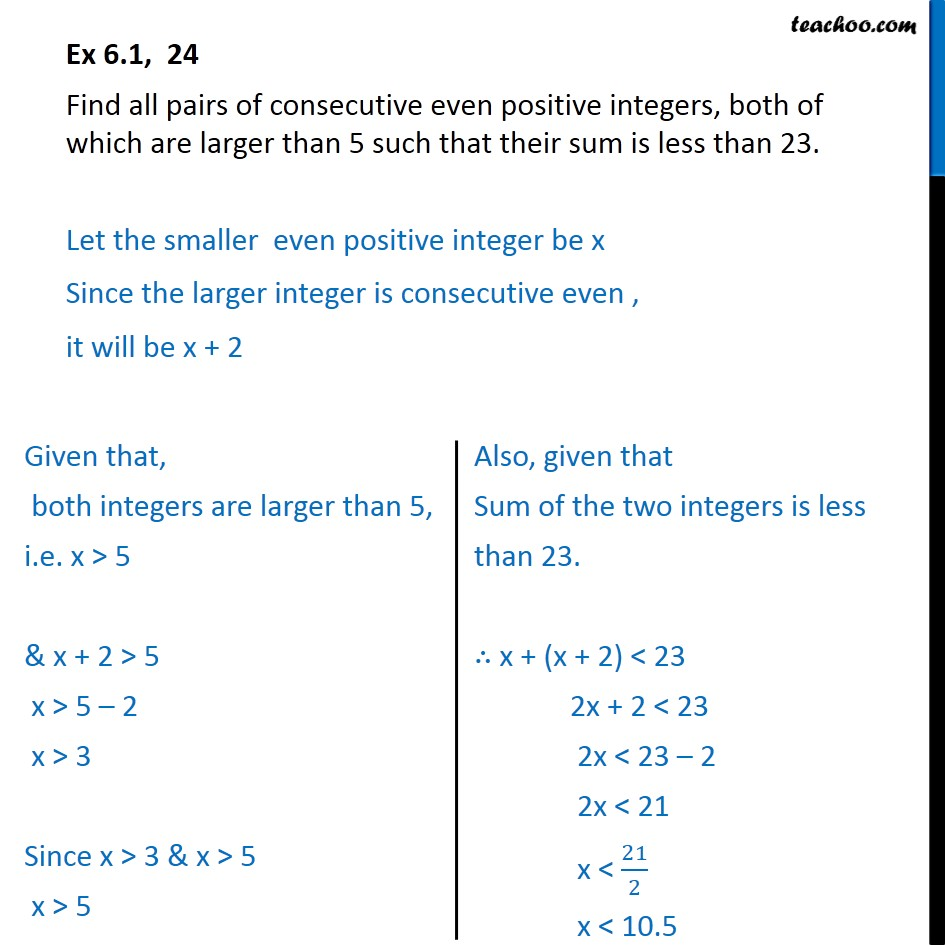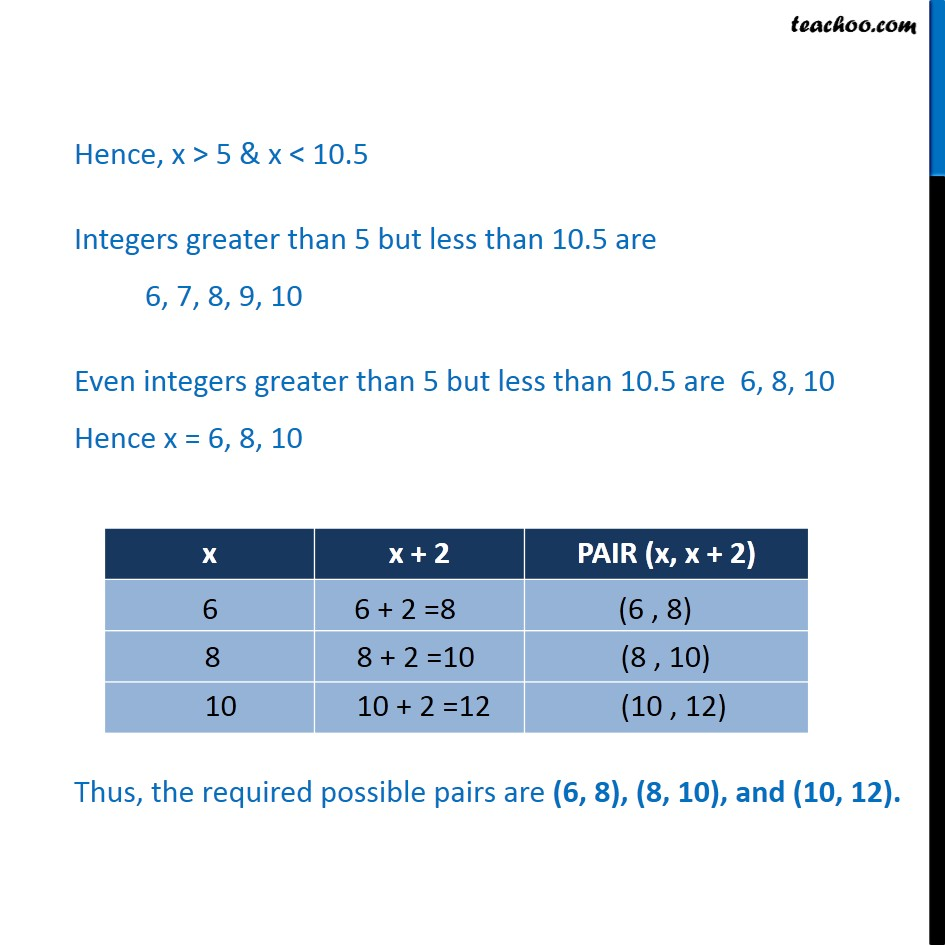Ex 6.1

Chapter 6 Class 11 Linear Inequalities (Term 2)
Serial order wise### Transcript

Ex 6.1, 24 Find all pairs of consecutive even positive integers, both of which are larger than 5 such that their sum is less than 23. Let the smaller even positive integer be x Since the larger integer is consecutive even , it will be x + 2 Hence, x > 5 & x < 10.5 Integers greater than 5 but less than 10.5 are 6, 7, 8, 9, 10 Even integers greater than 5 but less than 10.5 are 6, 8, 10 Hence x = 6, 8, 10